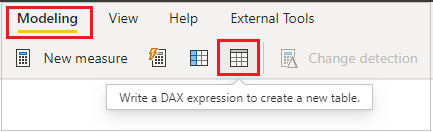Home » DAX » DAX – SUMMARIZE function

# DAX – SUMMARIZE functionReturns a summary table for the requested totals over a set of groups. It comes under Table Manipulation DAX Functions category.

#### Syntax:

```SUMMARIZE (<table>, <groupBy_columnName>, <groupBy_columnName> …,
<name>, <expression> …)```

#### Description:

 S no. Parameter Description 1 table Any DAX expression that returns a table of data. 2 groupBy_columnName (Optional) The qualified name of an existing column to be used to create summary groups based on the values found in it. This parameter cannot be an expression. 3 name The name given to a total or summarize column, enclosed in double quotes. 4 expression Any DAX expression that returns a single scalar value, where the expression is to be evaluated multiple times (for each row/context).

Step 1: Go to Modeling tab and click to TableCreate table

Step 2: DAX for Summarize table```Summarize Table =

SUMMARIZE(Orders,--- Table Name
Orders[Region], Orders[Product Category],--- Group by columns name
"Total Sale", SUM(Orders[Sales]),--- New column name with expression
"Total Profit", SUM(Orders[Profit]),--- New column name with expression
"Total Discount", SUM(Orders[Discount]),--- New column name with expression
"Total UnitPrice", SUM(Orders[Unit Price])--- New column name with expression

)```

OutputSummarize Table Output

Now you can perform other operations with this summarize table-

```East Sale = CALCULATE(
SUM('Summarize Table'[Total Sale]),
FILTER('Summarize Table', 'Summarize Table'[Region]="East"))```

#### Summarize DAX with Filter

```Summarize Table with filter =
SUMMARIZE(Orders,
Orders[Region], Orders[Product Category],
"Total Sale", SUMX(FILTER(Orders, Orders[Region] in {"East","Central"}), Orders[Sales]),
"Total Profit", SUM(Orders[Profit]),
"Total Discount", SUM(Orders[Discount]),
"Total UnitPrice", SUM(Orders[Unit Price])
)```

Output:Summarize with Filter

Hope you enjoyed the post. Your valuable feedback, question, or comments about this post are always welcome or you can leave us message on our contact form , we will revert to you asap.